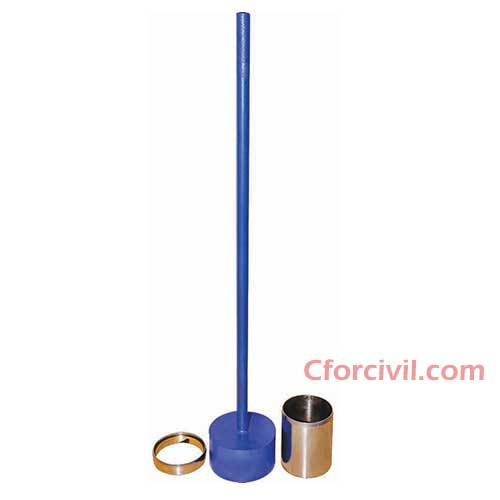# Calculation of Soil Compaction using Core Cutter MethodCalculation of  Soil Compaction using Core Cutter Method is discussed in this post. Soil Compaction using Core Cutter Method is use in construction field to determine the Field dry Density, Bulk Density and compaction of soil. It is use to identify the dry density of the compacted or dry fine grain soil which is free from the aggregates.

## Calculation of Soil Compaction using Core Cutter Method

For Calculation of Soil Compaction using Core Cutter Method some important parameters has to be derived. That important parameters are as follows,

• Volume of Core Cutter
• Weight of Core Cutter
• Weight of Core Cutter + Weight of Soil
• Soil Weight
• Bulk Density
• Moisture content or Water Content
• dry Density and
• Maximum dry Density### 1. Volume of Core Cutter (Vc)

The Volume of Core Cutter refers  to the volume of cylinder.

Volume of Core Cutter = Volume of cylinder.

Vc =π r² x h

(or)

Vc = πd²/4 x h

### 2. Weight of Core Cutter (Wc)

Measure the weight of Core Cutter from a calibrated Weighing balance machine and note as Wc. And it is taken in grams.

### 3. Weight of Core Cutter + Weight of Soil (Ws)

The weight of Core Cutter + weight of soil measures from Weighing balance machine and noted as Ws. And it is taken in grams.

### 4. Soil Weight (Wa)

The weight of soil (Wa) derives from ( Ws – Wc )

### 5. Bulk Density (Yb)

Bulk Density derives from the formula,

### (Yb)=(Ws-Wc / Vc)

where,

Wc = weight of Core Cutter,

Ws = weight of Core Cutter + weight of soil

Vc = Volume of Core Cutter

### 6. Moisture Content / Water Content (W) %

The Moisture Content / Water Content of the wet soil determines from Rapid Moisture Meter. The moisture/water content of wet soil derives from the formula,

### W= (M/100-M) x 100

The moisture/water content is taken in Percentage.

### (Yd)= (100 x Yb / 100 + w)

where,

Yb = Bulk Density,

W = Moisture Content / Water Content

### 8. Maximum Dry Density

The maximum value of Dry Density is taken and it is noted in grams.

### 9. Compaction Percentage

Compaction Percentage calculates by dividing the dry density by maximum dry density.

Cp % = Dry Density / Maximum Dry Density

## Method

 OBSERVATION SHEET DETERMINATION OF DRY DENSITY OF SOIL (CORE CUTTER METHOD) PROJECT:- LOCATION :- TESTED BY:- SI No Observation and Calculation Determination I II III 1 Volume of core cutter (Vc), cm3 2 Weight of core cutter (Wc), g 3 Weight of core cutter + Wet soil (Ws), g 4 Weight of wet soil (Ws-Wc), g 5 Bulk Density, Yb = ((Ws-Wc)/Vc)), g/cm3 6 (Rapid Moisture Meter) Water content (w),% 7 Dry Density, Yd = (Yb*100/(100+w)), g/cm3 8 Max. Dry Density, (g/cm3) 9 Compaction Percentage, % (DD/MDD) Average Dry Density, Yd =     %

##### 2 thoughts on “Calculation of Soil Compaction using Core Cutter Method”
1.sunil kp says:

Hi, I like your post really I have read first-time Thanks for sharing keep up the good work.

1.abbu riyaz says:

Thank you very much for your valuable comments and credits. Please like and share cforcivil as much as possible and make it popular among your circles. Help us to reach worldwide with our pieces of information and Civil Engineering knowledge